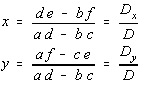# Cramer's Rule

 Let's solve a generic 2x2 system of linear equations.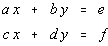To solve for x, let's eliminate the y's by multiplying the top equation by d and the bottom equation by -b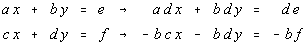When you add the two equations together, and solve for x, you get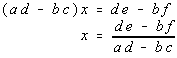Now, let's solve for y by eliminating the x's. Multiply the top equation by -c and the bottom equation by a.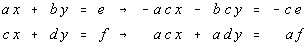When you add the two equations together, and solve for y, you get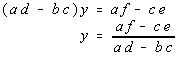Now, consider the following definitions The determinant D formed by taking the coefficients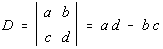The determinant Dx formed by taking the coefficient matrix and replacing the x's by the constants on the right hand side.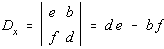The determinant Dy formed by taking the coefficient matrix and replacing the y's by the constants on the right hand side.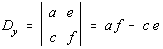Have you seen those determinants anywhere before? If not, then you've not been reading the lecture notes.